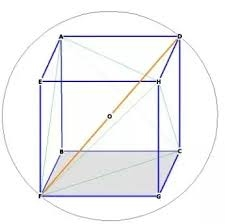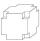# Eight

Eight small Christmas balls with a radius of 1 cm have the same volume as one large Christmas ball. What has a bigger surface: eight small balls, or one big ball?

x =  8

### Step-by-step explanation:Did you find an error or inaccuracy? Feel free to write us. Thank you!Tips to related online calculators
Tip: Our volume units converter will help you with the conversion of volume units.

#### You need to know the following knowledge to solve this word math problem:

We encourage you to watch this tutorial video on this math problem:

## Related math problems and questions:

• Plasticine ballPlasticine balls have radius r1=85 cm, r2=60 mm, r3=59 cm, r4=86 cm, r5=20 cm, r6=76 mm, r7=81 mm, r8=25 mm, r9=19 mm, r10=14 cm. For these balls
• BallsThree metal balls with volumes V1=71 cm3 V2=78 cm3 and V3=64 cm3 melted into one ball. Determine it's surface area.
• Big cubeCalculate the surface of the cube, which is composed of 64 small cubes with an edge 1 cm long.
• The ballThe ball has a radius of 2m. What percentage of the surface and volume is another sphere whose radius is 20% larger?
• Cube surface and volumeThe surface of the cube is 500 cm2, how much cm3 will be its volume?The surface of the sphere is 60 cm square. Calculate its radius; result round to tenth of cm.
• Cube cornersA small cube with an edge length of 2 cm was cut from each corner of a large cube with an edge length of 10 cm. How many cm3 was the body left from the big cube after cutting the small cubes?
• Area of a cubeCalculate the surface area of a cube if its volume is equal to 729 cubic meters.
• Cube surfce2volumeCalculate the volume of the cube if its surface is 150 cm2.
• Volume and areaWhat is the volume of a cube which has an area of 361 cm2?
• Cube and sphereCube with the surface area 150 cm2 is described sphere. What is sphere surface?
• Sphere A2VThe surface of the sphere is 241 mm2. What is its volume?
• Prism XThe prism with the edges of the lengths x cm, 2x cm, and 3x cm has volume 20250 cm3. What is the area of the surface of the prism?
• Iron sphereIron sphere has weight 100 kg and density ρ = 7600 kg/m3. Calculate the volume, surface, and diameter of the sphere.
• The cubeThe cube has a surface of 600 cm2. What is its volume?
• Cube 6Volume of the cube is 216 cm3, calculate its surface area.
• Thousand ballsWe have to create a thousand balls from a sphere with a diameter of 1 m. What will be their radius?